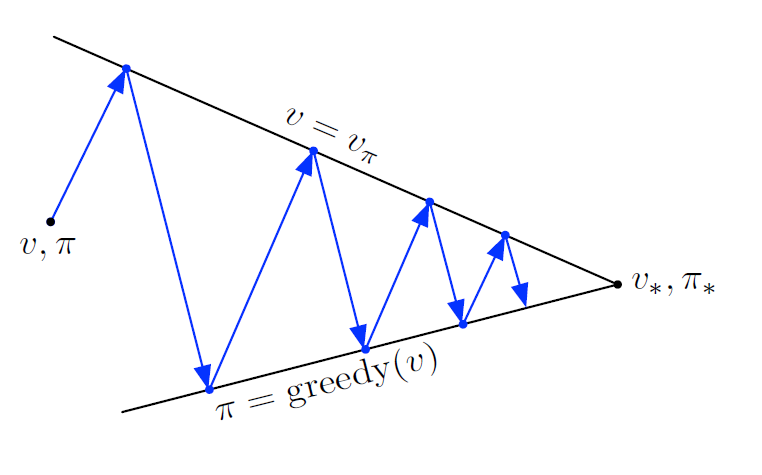# Fundamentals of Reinforcement Learning: Navigating Gridworld with Dynamic Programming

Source: Deep Learning on Medium# Introduction

Over the last few articles, we’ve covered and implemented the fundamentals of reinforcement learning through Markov Decision Process and Bellman Equations, learning to quantify values of specific actions and states of an agent within an environment. In this article, we’ll discuss Dynamic Programming and its role in Generalized Policy Iteration, a mutually reliant pair of processes that can self-optimize in order to identify the ideal trajectories within an environment to achieve maximum reward.

Dynamic programming (DP) is one of the most central tenets of reinforcement learning. Within the context of Reinforcement Learning, they can be described as a collection of algorithms that can be used to compute optimal policies iteratively, given a perfect model of the environment as a Markov Decision Process (MDP). Unfourtunately, their high computational expense coupled with the fact that most environments fail to reach this conditions of a perfect model, they are of limited use in practice. However, the concepts DP introduces lay the foundation for understanding other RL algorithms — In fact, most reinforcement learning algorithms can be seen as approximations to DP.

DP algorithms work to find optimal policies by iteratively evaluating solutions for Bellman equations, and then attempting to improve upon them by finding a policy that maximizes received reward. We’ve previously covered Bellman equations, advise the reader to consult our past articles for a deeper explanations.This sequence alternates until an optimal policy is identified.

DP algorithms primarily work for episodic, finite MDP environments, although it is possible to apply DP to continuous tasks via quantization. Recall that the value of a state is the expected reward of the state, which itself is a discounted sum of future rewards. After first initializing the value functions of a group of states arbitrarily, DP algorithms allow for the Bellman equation itself to be used as an update rule:

In practice, this is done by creating two arrays to hold the previous and present state-value functions. By using the values of the previous value function together with the DP algorithm, we can generate a new approximation for the value of that state. This is done one state at a time. It can be shown that states 𝑣𝑘 converges to the optimal 𝑣𝜋 as 𝑘 → ∞ under the same conditions that guarantee the existence of 𝑣𝜋, meaning that eventually these state value functions will stabilize.